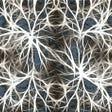# RL — Importance Sampling

Motivation

Importance sampling plays a key role in sampling inferencing and reinforcement learning RL. In RL, importance sampling estimates the value functions for a policy π with samples collected previously from an older policy π’. In simple term, calculating the total rewards of taking an action is very expensive. However, if the new action is relatively close to the old one, importance sampling allows us to calculate the new rewards based on the old calculation.

In specific, with the Monte Carlo method in RL, whenever we update the policy θ, we need to collect a completely new trajectory to calculate the expected rewards.

A trajectory can be hundreds in steps and it is awfully inefficient for one single update. With importance sampling, we just reuse the old samples to recalculate the total rewards. However, when the current policy diverges from the old policy too much, the accuracy decreases. Therefore, we do need to resync both policies regularly.

In other situations. we use importance sampling to rewrite the Policy Gradient equation and use them to create new solutions.

For example, we can reposition our optimization with constraints of not going too far in changing the policy. We formalize a trust-region concept which we believe the approximation is still accurate enough for those new policies within the region.

By not making too aggressive moves, we have better assurance that we don’t make bad changes that spoils the training progress. As we keep improving the policy, we locate the optimal solution.

What is Importance sampling?

Importance sampling is a technique of estimating the expected value of f(x) where x has a data distribution p. However, Instead of sampling from p, we calculate the result from sampling q.

i.e. we use the sampling distribution q to estimate the expected value for p using:

For this to work q(xi) cannot be zero if the corresponding p(xi) is not zero. Let’s demonstrate it with an example.

Let’s first calculate Ep[f(x)] and Eq[f(x)]. They should be different. In the following example, we calculate Eq[f(x)] with distribution p.

Now, we re-weight the expected value with distribution p.

Now, we show that we can use different sampling distributions to compute an expected value. In RL, we reuse sampling results from an old policy to refine the current policy.

In sampling inference, p(x) is already modeled and calculating p(x) for each x is not hard. However, there is a misconception that if we know the equation of p, we know how to create samples representing the distribution p easily. This is true for well-known distributions only. In general, it is not true. So for sampling inference, we use a more tractable distribution q to generate samples instead.

Optimal Importance Sampling

The accuracy of the expected value increases as the number of samples increase. So what will be the best sampling distribution in minimizing the variance of our estimation?

To compute the expectation of f.

We can use the importance sampling with sampling distribution q.

For the estimation to have the minimum variance, the sampling distribution q should be:

Intuitively, it means if we want to reduce the variance of our estimation, we want to sample data points with higher rewards. For example, the diagram on the right sampled data proportional to the reward value and therefore, it will produce estimations that have a smaller variance. (i.e. better estimations)

# Normalized importance sampling

The method above is called unnormalized importance sampling. Many ML models belong to the Bayesian network or Markov Random Field (MRF). In a Bayesian network, p is easy to compute. But how can we extend it to other models like MRF which only the unnormalized distribution is easy to compute?

Let’s reformulate the equation based on unnormalized p instead.

We compute rᵐ with the unnormalized distribution and then used the total sum of rᵐ to normalize the distribution. This avoids computing the usual normalization factor Z which is usually hard to compute. This is called the normalized importance sampling.

But this approach does come with a price. Unnormalized importance sampling is unbiased while the normalized importance is biased. However, normalized importance sampling is likely has less variance.

--

--

--

## More from Jonathan Hui

Deep Learning

Love podcasts or audiobooks? Learn on the go with our new app.

## Day 2: Observe this given Dataset classify into what type of ML problem it is and train an ML…## Machine Learning adoption for any organization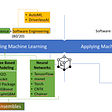## How to create your own deep learning framework using only Numpy## Multi-Task Learning in Language Model for Text Classification## What is Convolutional Neural Network(CNN) Architecture in Computer Vision?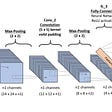## Neural Networks with Google CoLaboratory | Machine Learning Getting started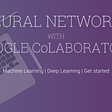Deep Learning

## Statistics (III) ANOVA in Data Science & Machine Learning## What is inductive bias?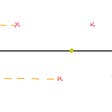## Lottery ticket hypothesis in deep learning## Intuition to Neural Network embeddings